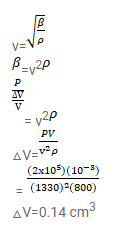# Find the change in the volume ofQuestion:

Find the change in the volume of $1.0$ litre kerosene when it is subjected to an extra pressure of $2.0 \times 10^{5} \mathrm{~N} \mathrm{~m}^{-2}$ from the following data. Density of kerosene $=800 \mathrm{~kg} \mathrm{~m}^{-3}$ and speed of sound in kerosene $=1330 \mathrm{~ms}^{-1}$.

Solution: